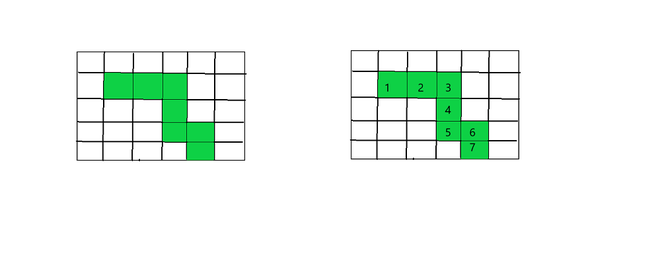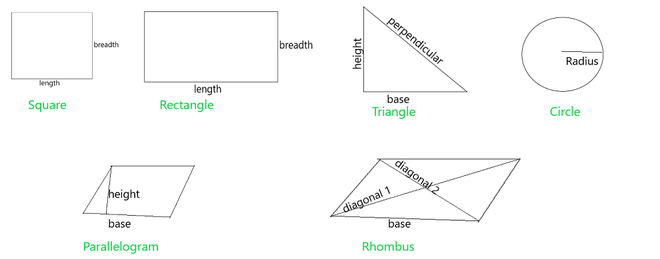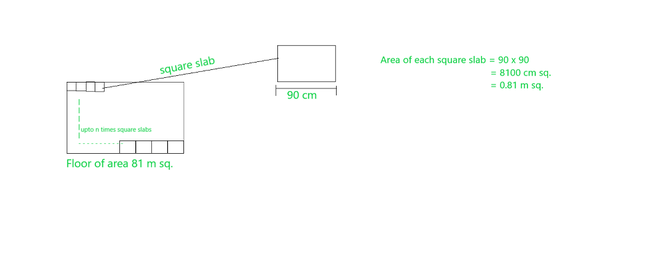# How many square slabs each with side 90cm are needed to cover a floor of area 81m?

• Last Updated : 21 Nov, 2021

The area is defined as the amount of space occupied by a 2-dimensional shape. In a different way, it is the quantity that calculates the number of unit squares that cover the surface of any closed/bounded figure. The standard unit of area is square units which are generally represented as square meter, square feet, square inch, square centimeter, etc.

The closed shapes are bounded and the area is calculated with the help of its length and width. Length is single in direction means unidirectional and its unit is meter (m), centimeter (cm), etc. the area of any figure is a two-dimensional quantity i.e. the product of length and width. Hence, it is measured in square units like a square centimeter or (cm2), square meter (m2) square feet or (ft2), etc.

### Measuring Area of any figure

Let’s learn this concept with the help of grids which are in the shape of a square, each grid is made up of many squares of sides 1 unit by 1 unit. hence the area is 1 square unit of each square. therefore it can be said that each square is 1 square unit Look at the figure shown below. now we will find the area of the below shape with the help of the grid.The area of the colored region is the number of shaded unit squares

From the figure, there are 7 colored squares therefore the area of the shape is 7 square units. because each box are one square unit, and there is 7 colored square in total therefore, its square is 7 square units.

Area of different shapes

For different shapes, different formulae are defined and they are derived based on the properties a shape has, for instance, a square has all the equal and therefore, the area of the square can simply be the square of the side. Below are the shapes have given and let’s take a look at these formulae for different shapes,### How many square slabs each with a side of 90 cm are needed to cover a floor of area 81 m2?

Solution:Floor of area 81 m sq. and a slab of length 90 cm

The side of square slab = 90 cm

Area of square slab = 90 × 90

a = 8100 cm2

a = 8100/100 × 100 m2

a   = 0.81 m2

Area of floor, A = 81 m2

Number of slabs that will cover the floor =  Area of square slab/ Area of the floor

= 81 / 0.81

= 100

​So, there are 100 slabs needed to cover a floor of area 81 m2.

### Sample problem

Question 1: Calculate the area of a square, if its side is 4 cm.

Solution:

Area of Square = side × side

= 4 × 4 = 16 cm2

Question 2: Calculate the side of the square, if its area is 64m2.

Solution:

Area of Square = 64 m2

Side × side = 64

Side = √64

Side = 8 m

Question 3: Find the area of a square if its perimeter is 48cm.

Solution:

Given, perimeter of square = 48 cm

4 × side = 48

Side   = 48/4

Side = 12 cm

Area of square = side × side

= 12 × 12

= 144 cm2

Hence, area of square is 144 cm2

Question 4: If, the square of side 6 cm and the rectangle of dimensions 4 cm x s cm, if both have same areas calculate the value of s.

Solution:

Given side of square = 6 cm

Then, area of square A’ = 6 × 6 = 36 cm2

Area of rectangle A” = 4 × s cm2.

According to question

Area of rectangle (l × b) = area of square (side × side)

4 × s =  36

s = 36/4

s = 9 cm

Therefore value of s is 9 cm

Question 5: If the side of the square becomes double, what will happen to its area.

Solution:

Let, the side of square be s

Now the Area of square = side × side

A = s cm2

If the side s doubles then, New side

s’ = 2s

Area of new square = side × side= s’ × s’

A’ = 2s × 2s = 4s2

A’ = 4 × A (A = s2)

From the above result, If the square side is doubled then area of square will become four times.

Question 6: If the area of a rectangle is 120 square cm and one of its dimensions is 15cm, calculate the other.

Solution:

Given,

length of rectangle, l = 15 cm

Area of rectangle, A = 120 cm2.

Area of rectangle = length × breadth

120 = 15 × b

b = 120/15

b = 8 cm.

Hence, other dimension of rectangle is 8cm.

My Personal Notes arrow_drop_up